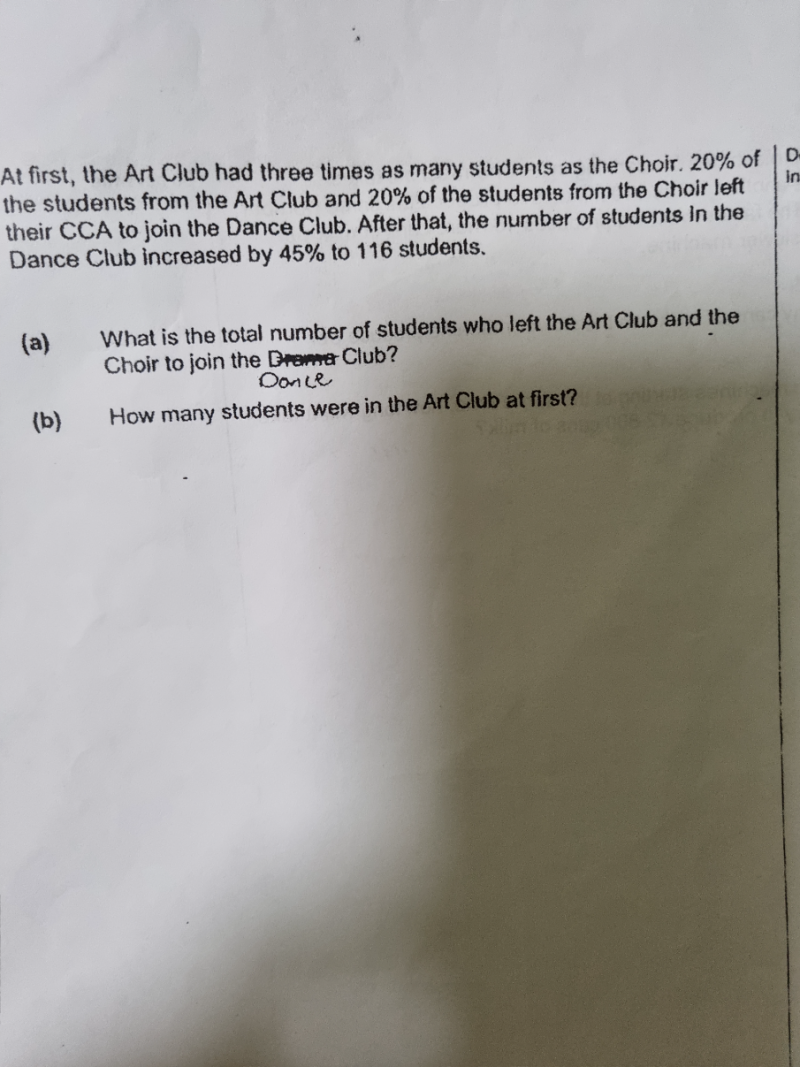# QuestionThank you.

Art         [u][u][u]

Choir     [u]

Dance increased 45% to 116

(a)  145% -> 116

45% -> (116/145)   x 45  = 36

Hence total number of students who left the Art Club and the Choir is 36

(b)   20% = 1/5

(1/5)(3u) + (1/5)(u) = 36   <——— x 5 on both sides

3u + u = 180

4u = 180   => u = 180/4 = 45

Hence number of students in the Art club at first = 3u = 3 x 45 = 135